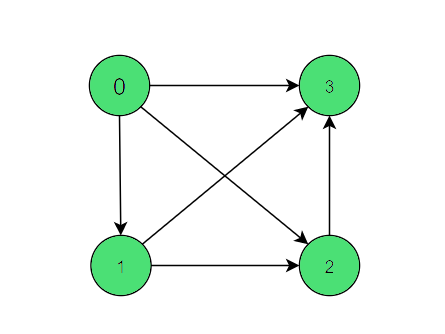# Check if incoming edges in a vertex of directed graph is equal to vertex itself or not

Given a directed Graph G(V, E) with V vertices and E edges, the task is to check that for all vertices of the given graph, the incoming edges in a vertex is equal to the vertex itself or not.

Examples:

Input:Output: Yes
Explanation:
For vertex 0 there are 0 incoming edges, for vertex 1 there is 1 incoming edge. Same for vertex 2 nd 3.

## Recommended: Please try your approach on {IDE} first, before moving on to the solution.

Approach: The idea is to traverse adjacency list for every vertex, and increment the count of edges of every vertex that has an incoming edge from i. Repeat the steps for every vertex and then check the in degrees for all the vertices equal to vertex value or not.

Below is the implementation of the above approach:

## C++

 `// C++ implementation to check if the ` `// incoming edges in a vertex of directed ` `// graph is equal to the vertex itself or not ` ` `  `#include ` `using` `namespace` `std; ` ` `  `// A utility function to ` `// add an edge in an ` `// directed graph ` `void` `add_edge(vector<``int``> adj[], ` `              ``int` `x, ``int` `y) ` `{ ` `    ``adj[x].push_back(y); ` `} ` ` `  `// Function to check that given graph ` `// in-degree value equal to vertex value ` `bool` `Indegree(vector<``int``> adj[], ``int` `v) ` `{ ` `    ``// Create array indeg ` `    ``// intialized to zero ` `    ``int` `indeg[v] = { 0 }; ` ` `  `    ``// Traversing across all ` `    ``// vertex to compute ` `    ``// in degree value ` `    ``for` `(``int` `i = 0; i < v; i++) { ` `        ``for` `(``int` `j = 0; j < adj[i].size(); j++) { ` `            ``indeg[adj[i][j]]++; ` `        ``} ` `    ``} ` ` `  `    ``// check in degree value ` `    ``// equal to vertex value ` `    ``for` `(``int` `i = 0; i < v; i++) { ` `        ``if` `(i == indeg[i]) ` `            ``continue``; ` `        ``else` `            ``return` `false``; ` `    ``} ` `    ``return` `true``; ` `} ` ` `  `// Driver code ` `int` `main() ` `{ ` ` `  `    ``int` `v = 4; ` ` `  `    ``// To store adjacency list of graph ` `    ``vector<``int``> adj[v]; ` `    ``add_edge(adj, 0, 1); ` `    ``add_edge(adj, 1, 2); ` `    ``add_edge(adj, 0, 2); ` `    ``add_edge(adj, 0, 3); ` `    ``add_edge(adj, 1, 3); ` `    ``add_edge(adj, 2, 3); ` ` `  `    ``if` `(Indegree(adj, v)) ` `        ``cout << ``"Yes"``; ` ` `  `    ``else` `        ``cout << ``"No"``; ` `} `

## Python3

 `# Python3 implementation to check if the ` `# incoming edges in a vertex of directed ` `# graph is equal to the vertex itself or not ` ` `  `# A utility function to ` `# add an edge in an ` `# directed graph ` `def` `add_edge(adj, x, y): ` `     `  `    ``adj[x] ``=` `adj[x] ``+` `[y] ` ` `  `# Function to check that given graph ` `# in-degree value equal to vertex value ` `def` `Indegree(adj, v): ` ` `  `    ``# Create array indeg ` `    ``# intialized to zero ` `    ``indeg ``=` `[``0``] ``*` `v ` ` `  `    ``# Traversing across all ` `    ``# vertex to compute ` `    ``# in degree value ` `    ``for` `i ``in` `range``(v): ` `        ``for` `j ``in` `range``(``len``(adj[i])): ` `            ``indeg[adj[i][j]] ``+``=` `1` ` `  `    ``# Check in degree value ` `    ``# equal to vertex value ` `    ``for` `i ``in` `range``(v): ` `        ``if``(i ``=``=` `indeg[i]): ` `            ``continue` `        ``else``: ` `            ``return` `False` ` `  `    ``return` `True` ` `  `# Driver code ` `if` `__name__ ``=``=` `'__main__'``: ` ` `  `    ``v ``=` `4` ` `  `    ``# To store adjacency list of graph ` `    ``adj ``=` `[[]] ``*` `4` `    ``add_edge(adj, ``0``, ``1``) ` `    ``add_edge(adj, ``1``, ``2``) ` `    ``add_edge(adj, ``0``, ``2``) ` `    ``add_edge(adj, ``0``, ``3``) ` `    ``add_edge(adj, ``1``, ``3``) ` `    ``add_edge(adj, ``2``, ``3``) ` ` `  `    ``if``(Indegree(adj, v)): ` `        ``print``(``"Yes"``) ` `    ``else``: ` `        ``print``(``"No"``) ` ` `  `# This code is contributed by Shivam Singh `

Output:

```Yes
```

Time Complexity: O(V + E)
Auxiliary Space Complexity: O(V)

Attention reader! Don’t stop learning now. Get hold of all the important DSA concepts with the DSA Self Paced Course at a student-friendly price and become industry ready.

My Personal Notes arrow_drop_upCheck out this Author's contributed articles.

If you like GeeksforGeeks and would like to contribute, you can also write an article using contribute.geeksforgeeks.org or mail your article to contribute@geeksforgeeks.org. See your article appearing on the GeeksforGeeks main page and help other Geeks.

Please Improve this article if you find anything incorrect by clicking on the "Improve Article" button below.

Article Tags :
Practice Tags :

Be the First to upvote.

Please write to us at contribute@geeksforgeeks.org to report any issue with the above content.